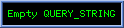To download: Right-click on the file, then select 'Save Target As...' Professor K. Krishnamoorthy [Dept of Mathematics, UL Lafayette, Lafayette, Louisiana, USA] Updated on 12/03/06 StatCalc.zip Description: StatCalc is a PC calculator that computes table values of many commonly used statistical distributions. It also computes moments, and many other statistics; see the table of content. Download and click on setup.exe. StatCalc also comes with help files.   Operating Systems: Windows 7, XP, 2000; Windows 8. Disk space : 10 MB. Right click the link on the left, and select “save target as.” Click on setup.exe. Admin privileges is not required to install the software. In case you encounter any installation problems,  download StatCalc.zip, and extract the files in the same directory. Fortran Programs... Comments to krishna@louisiana.edu Fortran program to evaluate the cdf of the noncentral t [Benton, D. and Krishnamoorthy, K. (2003). Computing discrete mixtures of continuous distributions: noncentral chisquare, noncentral t and the distribution of the square of the sample multiple correlation coefficient. Computational Statistics and Data Analysis, 43, 249-267] Fortran program to evaluate the cdf of the noncentral chi-square [ Benton, D. and Krishnamoorthy, K. (2003)] Fortran program: computes the p-value for testing the difference between proportions from two finite populations. [Krishnamoorthy, K. and Thomson, J. (2002). Hypothesis testing about proportions in two finite populations. The American Statistician, 56, (2002), 215-222.] Fortran program: computes the power of the test (3) for the difference between proportions from two finite populations. [Krishnamoorthy, K. and Thomson, J. (2002)] Fortran program: computes the p-value for testing the difference between two Poisson means. [Source: Krishnamoorthy, K and Thomson, J. (2004) A more powerful test for comparing two Poisson means. Journal of Statistical Planning and Inference, 119, 249-267] Fortran program: computes the power of the unconditional test for the difference between two Poisson means. [Krishnamoorthy, K and Thomson, J. (2004) A more powerful test for comparing two Poisson means. Journal of Statistical Planning and Inference, 119, 249-267] SAS code Fortran program: computes confidence limits for the mean of a lognormal distribution. [Krishnamoorthy, K. and Mathew, T. (2003) Inferences on the means of lognormal distributions using generalized p-values and generalized confidence intervals. Journal of Statistical Planning and Inference, (2003), 115, 103 – 121] Help7.pdf 8. Power: Lognormal mean SAS code Computes the power of the test in (7). For a given mean and standard deviation of the logged data, and the sample size, this program computes the power of the test in (7). Help8.pdf 9. Lognormal: Two-sample SAS code Computes the p-value for testing the difference between two lognormal means. Also, computes the confidence interval for the difference between the means. Help9.pdf 10. Lognormal: Power for Two-sample Test SAS code Computes the power of the test (9) for testing the difference between two lognormal means. Help10.pdf 11. One-sided limits for exceedance probabilities Computes the one-sided limits for the exceedance probabilities assuming random effects model. See Krishnamoorthy, K. Mathew, T.  and Ramachandran, G. (2007). Upper limits for the exceedance probabilities in one-way random effects model.  Annals of Occupational Hygiene, 51, 397-406. 12. R program                      EXPOSURE_MDLS.r; help file Krishnamoorthy, K. and Zhao Zu (2011). Confidence Limits for Lognormal Percentiles and for Lognormal Mean based on Samples with Multiple Detection Limits. Annals of Occupational Hygiene, 55,  495-509.  Krishnamoorthy, K., Mathew, T. and Xu, Z. (2014). Standardized likelihood inference for the mean and percentiles of a lognormal  distribution  based on samples with multiple detection limits. Journal of Environmental Statistics, 6, 1–18. 13. GammaInfer.r This R program computes confidence intervals for gamma parameters and mean, CIs for the difference between two means, difference between two shape parameters. Also, calculates the p-values for testing the mean and parameters, comparing two means, two scale parameters and for comparing two shape parameters. Krishnamoorthy, K. and Luis, N. (2014). Small sample inference for gamma distributions: one- and two-sample problems. Environmetrics, 25, 107-126.You arevisitor ! ... since March 3, 2001